# node-red-contrib-calc 1.0.6

A Node-Red node to perform basic mathematical calculations

`npm install node-red-contrib-calc`

A Node-Red node to perform basic mathematical calculations

## Install

Run the following npm command in your Node-RED user directory (typically ~/.node-red):

``````npm install node-red-contrib-calc
``````

For more advanced mathematical operations, please have a look at the node-red-contrib-statistics node.

## Support my Node-RED developments

Please buy my wife a coffee to keep her happy, while I am busy developing Node-RED stuff for you ...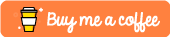## Usage

Four steps are involved to execute a mathematical calculation via this node:

1. An input data is send to this node with a number or an array of numbers in the input message. By default the data will arrive via `msg.payload`, but another input message field can be selected: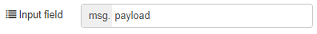How many numbers should be available in the input message, depends on the operation type:

• Most operations require only a single input number. For example a single input number `-3` is enough to calculate the absolute value. All operations like this one will also accept an array of numbers as input data. In that case the same operation will be executed on every number in the array! For example the absolute value of array `[-7, -3, -9, -12]` will result in `[7, 3, 9, 12]`.
• Some other operations require always an array of input numbers. For example an array of minimum 2 input numbers `[2, 3]` is required to multiply, but the result will be a single output number `6`.
• A few operations require a fixed-length array of input numbers. For example X to the power of Y requires an array of two input numbers.
2. The node will execute the requested operation on the input data.

3. If required, the result of the calculation will be rounded to the specified number of decimals (or truncated).

4. The result of the calculation will be stored in the output message. The result can be a single output number or an array of output numbers. By default the data will be put in `msg.payload`, but another output message field can be selected: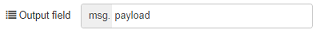# Example flow

The following flow shows how to search the maximum number from an array of injected numbers: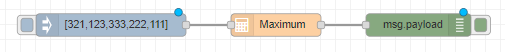``````[{"id":"b6bc5399.8385e","type":"calculator","z":"4142483e.06fca8","name":"","inputMsgField":"payload","outputMsgField":"payload","operation":"max","constant":"","round":false,"decimals":0,"x":640,"y":3060,"wires":[["4c297cba.7585a4"]]},{"id":"e5a3b930.003428","type":"inject","z":"4142483e.06fca8","name":"","topic":"","payload":"[321,123,333,222,111]","payloadType":"json","repeat":"","crontab":"","once":false,"onceDelay":0.1,"showConfirmation":false,"confirmationLabel":"","x":420,"y":3060,"wires":[["b6bc5399.8385e"]]},{"id":"4c297cba.7585a4","type":"debug","z":"4142483e.06fca8","name":"","active":true,"tosidebar":true,"console":false,"tostatus":false,"complete":"false","x":830,"y":3060,"wires":[]}]
``````

## Operations

Following operations are available:

• Average (avg): average of all the numbers in the input array.

Input = `[1, 2, 3, 4]` => Output = `2.5`

• Maximum (max): get the number with the highest value from an array of numbers.

Input = `[1, 2, 3, 4]` => Output = `4`

• Minimum (min): get the number with the lowest value from an array of numbers.

Input = `[1, 2, 3, 4]` => Output = `1`

• Increment (inc): add 1 to the number.

Input = `4` => Output = `5`

Input = `[1, 2, 3, 4]` => Output = `[2, 3, 4, 5]`

• Decrement (dec): subtract 1 from the number.

Input = `4` => Output = `3`

Input = `[1, 2, 3, 4]` => Output = `[0, 1, 2, 3]`

• Integer part (trunc): truncate (trunc) the number to the integer part.

Input = `4.6` => Output = `4`

Input = `[1.3, 2.5, 3.7]` => Output = `[1, 2, 3]`

• Round upwards (ceil): round the number upwards (ceil) to the nearest integer.

Input = `4.6` => Output = `5`

Input = `[1.3, 2.5, 3.7]` => Output = `[2, 3, 4]`

• Round downwards (floor): round the number downwards (floor) to the nearest integer.

Input = `4.6` => Output = `4`

Input = `[1.3, 2.5, 3.7]` => Output = `[1, 2, 3]`

• Nearest integer (round): rounds the number to the nearest integer.

Input = `4.6` => Output = `5`

Input = `[1.3, 2.5, 3.7]` => Output = `[1, 3, 4]`

• Round decimal places (rdec): round the number at a specified number of decimal places (from an array of two numbers).

Input = `[1.23456, 3]` => Output = `[1.235]`

• Sum (sum): sum of the all the numbers in the array.

Input = `[1, 2, 3, 4]` => 1 + 2 + 3 + 4 => Output = `10`

• Subtract (sub): subtraction of the all the numbers in the array.

Input = `[3, 2, 1]` => 3 - 2 - 1 => Output = `0`

• Truncate decimal places (tdec): truncate the number at a specified number of decimal places (from an array of two numbers).

Input = `[1.56789, 3]` => Output = `[1.567]`

• Multiply (mult): multiply all the numbers in the array.

Input = `[3, 2, 1]` => 3 * 2 * 1 => Output = `6`

• Divide (div): division of all the numbers in the array.

Input = `[3, 2, 1]` => 3 : 2 : 1 => Output = `1.5`

• Modulus (mod): get the remainder of the division of the two numbers in the array.

Input = `[3, 2]` => 3 % 2 => Output = `1`

• Absolute value (abs): absolute value (abs) of the number.

Input = `-4` => Output = `4`

Input = `[-3, -5, -7]` => Output = `[3, 5, 7]`

• Random (rand): a random number between 0 and 1. The input value will not be checked, since it is not required to calculate the output value. When the input is an array of N length, then the output will also be an array containing N random numbers.

Input = `x` => Output = `0.xxxxx`

Input = `[x, x, x]` => Output = `[0.xxxxx, 0.xxxxx, 0.xxxxx]`

• Random between min and max (randb): a random number between a minimum value and a maximum value, which both need to be specified in the input array.

Input = `[3, 8]` => Output = `3` or `4` or `5` or `6` or `7` or `8`

• Random from array (randa): a random number picked from an array of possible values.

Input = `[3, 5, 8]` => Output = `3` or `5` or `8`

• Length of array (len): the length of the input array. The input values will not be checked, since it is not required that the array only contains numbers.

Input = `[7, "text", true, 8]` => Output = `4`

• Sort ascending (sorta): sort the input array (containing numbers) ascending, i.e. from low to high.

Input = `[9, 8, 7]` => Output = `[7, 8, 9]`

• Sort descending (sortd): sort the input array (containing numbers) descending, i.e. from high to low.

Input = `[7, 8, 9]` => Output = `[9, 8, 7]`

• Create range (range): create an array of numbers, between the two numbers (minimum and maximum) in the array.

Input = `[2, 8]` => Output = `[2, 3, 4, 5, 6, 7, 8]`

Input = `[2.1, 8.6]` => Output = `[2.1, 3.2, 4.2, 5.2, 6.2, 7.2, 8.2]`

• Get distance (dist): get the distance between the numbers in the array, i.e. the range between the maximum and minimum number.

Input = `[2, 9, 1, 8, 3]` => Output = `8`

• X to the power of y (pow): xy from an array of two numbers.

Input = `[2, 3]` => 23 => Output = `8`

• E to the power of x (exp): value of Ex, where E is Euler's number (approximately 2.7183).

• Cubic root (cbrt): cubic root (x3) of the number.

• Natural logarithm (log): natural logarithm base E of the number.

• Logarithm (log10): logarithm base 10 of the number.

• Arccosine (acos): arccosine (acos) value of the number.

• Hyperbolic arccosine (acosh): hyperbolic arccosine of the number.

• Arcsine (asin): arcsine of the number in radians.

• Hyperbolic arcsine (asinh): hyperbolic arcsine of the number.

• Arctangent (atan): arctangent of the number, as a numeric value between -PI/2 and PI/2 radians.

• Hyperbolic arctangent (atanh): hyperbolic arctangent of the number.

• Cosine (cos): cosine of the number in radians.

• Hyperbolic cosine (cosh): hyperbolic cosine of the number.

• Sine (sin): sine of the number in radians.

• Hyperbolic sine (sinh): hyperbolic sine of the number.

• Square root (sqrt): square root of the number.

• Tangent (tan): tangent of an angle.

• Hyperbolic tangent (tanh): hyperbolic tangent of the number.

## Message based operation

When no operation is specified in the config screen, the operation needs to be specifiedin the `msg.operation` field of the input message. In the above list of available operations, the operation code is specified between angle brackets.

For example to calculate the 'Cubic root' of a number, the `msg.operation` field should contain value `cbrt`.

## (Optional) constant values

Almost all operations allow an optional constant to be used: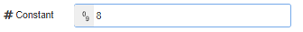When a number is entered in this field, it will automatically be added at the end of the input array. When the input is a number, this node will convert it automatically to an array (containing both the number and the constant value).

Some examples with constant value `3`:

• Round constant number of decimal places:

Input = `1.23456` => Internal = `[1.23456, 3]` => Output = `[1.234]`

• X to a constant power:

Input = `2` => Internal = `[2, 3]` => Output = `8`

• Sum (sum): sum of the all the numbers in the array.

Input = `[7, 2, 5]` => Internal = `[7, 2, 5, 3]` => Output = `17`

## Round result to ... decimals

When selected, the output number(s) will be rounded to the specified number of decimals. This rounding can be applied to the result of any selected operation. Note that the "Round decimal places" operation can be used instead, when it is only required to round the numeric values in the input message.

## Truncate result to ... decimals:

When selected, the output number(s) will be truncated to the specified number of decimals. This truncating can be applied to the result of any selected operation. Note that the "Truncate decimal places" operation can be used instead, when it is only required to truncate the numeric values in the input message.

#### Node Info

Version: 1.0.6
Updated 17 hours ago
Rating: 4.2 5

#### Actions

Rate:

528 in the last week

• calculator

• node-red
• math
• mathematics
• calculate
• min
• max
• round
• trunc
• min
• max
• average